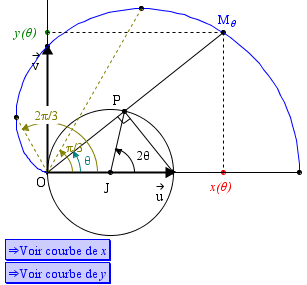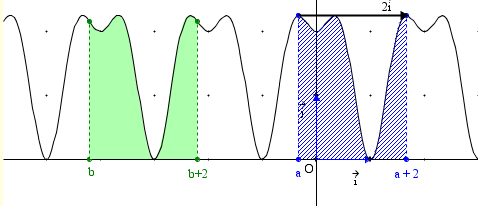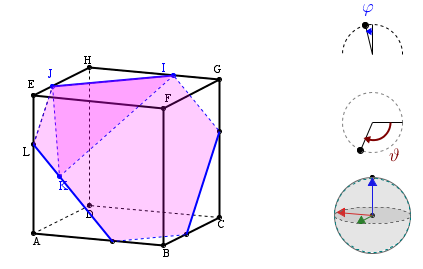Search IntMath
Close

# MathGraph32

By Murray Bourne, 30 Apr 2013

MathGraph32 is a great free tool for exploring 2D and 3D math concepts.

(It's been around for a while, but I only recently discovered it.)

There is a download version as well as an online Java-based version.

UPDATE (May 2018): MathGraph32 is now Javascript-only, meaning it is capable of running on most operating systems and most browsers.

According to the site, MathGraph32 is an:

Open source cross-platform software of geometry, analysis and simulation.

It was developed by French-speaking mathematician, Yves Biton. Some of the examples are in French, but it's quite easy to see what is going on. (There is an English version of the program).

## Screen Shots

Here are some examples from MathGraph32 (images by them).You can explore several examples (some for "teaching purposes"), and there are tutorials that help explain the use of the applet.

It's well worth checking out!

### Comment Preview

HTML: You can use simple tags like <b>, <a href="...">, etc.

To enter math, you can can either:

1. Use simple calculator-like input in the following format (surround your math in backticks, or qq on tablet or phone):
a^2 = sqrt(b^2 + c^2)
(See more on ASCIIMath syntax); or
2. Use simple LaTeX in the following format. Surround your math with $$ and $$.
$$\int g dx = \sqrt{\frac{a}{b}}$$
(This is standard simple LaTeX.)

NOTE: You can mix both types of math entry in your comment.